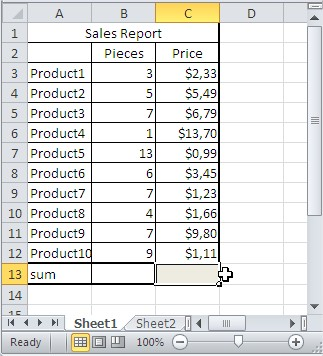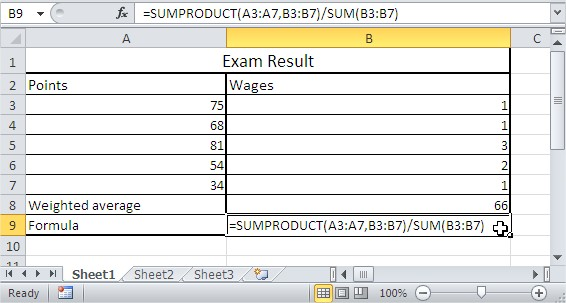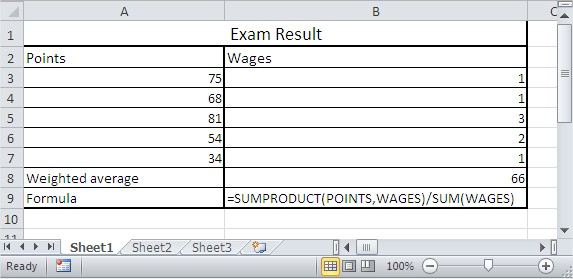#### How to use Sumproduct Function in Excel

In this lesson you can learn how to use SUMPRODUCT function in Excel.

SUMPRODUCT is the most important (next to SUM) Excel function used in operations research. This function multiplies in the given arrays, and returns the sum of those products.

SUMPRODUCT stands for “Sum of Products”. It is a function that takes two or more arrays as arguments and returns the sum of the products of corresponding elements in the arrays.

## Sumproduct function syntax

Syntax is:

=SUMPRODUCT(array1,[array2],[array3],…)

## Sumproduct basics

=SUMPRODUCT (A1:C1,A2:C2) means A1*A2+B1*B2+C1*C2

=SUMPRODUCT(A4:A7,B4:B7) means A4*B4+A5*B5+A6*B6+A7*B7

=SUMPRODUCT(A9:B11,D9:E11) means A9*D9+A10*D10+A11*D11+B9*E9+B10*E10+B11*E11

=SUMPRODUCT(E9:E11,G9:G11) means E9*G9+E10*G10+E11*G11

Notes:

(array1, [array2], [array3], …) is from 2 to 30 arrays whose elements you multiply and add. Arrays must be in the same dimensions (ranges of cells must have the same number of rows and columns). If it is not, the function SUMPRODUCT returns the #VALUE! error.

## How does the SUMPRODUCT function work?

The SUMPRODUCT function works by multiplying the corresponding cells in the two arrays and then summing the products. For example, if you have two arrays, A and B, and you want to calculate the sum of the products of the elements in the first row of A and the first column of B, you would use the following formula:

=SUMPRODUCT(A1:A2, B1:B2)

This formula would return the sum of 21 and 32, which is 8.

## Examples of Sumproduct function

Here are some examples of how to use the SUMPRODUCT function in Excel:

### Sum of sales in report

You have sales report. In B column there is count of pieces sold. In C column there are prices. How to calculate sum of sales?Formula here is: =SUMPRODUCT(B3:B12,C3:C12)

Result is \$223.08.

### Calculating weighted average with Sumproduct function

To calculate weighted average of exam’s result in Excel use below formula:

=SUMPRODUCT(A3:A7,B3:B7)/SUM(B3:B7)As you see points in 5th row are the most important.

### SUMPRODUCT and name ranges

Let’s use the same example. You can name ranges and use them instead of ranges. I named ranges A3:A7 as POINTS and B3:B7 as WAGES.

Now I can change formula

from =SUMPRODUCT(A3:A7,B3:B7)/SUM(B3:B7)

to =SUMPRODUCT(POINTS,WAGES)/SUM(WAGES)## Tips for using the SUMPRODUCT function in Excel

The SUMPRODUCT function is a volatile function, which means that it recalculates its value whenever the workbook is recalculated. This can be useful if you want to keep the sum of the products up to date, but it can also be a problem if you are using the SUMPRODUCT function in a formula that should not be recalculated every time the workbook is opened.

The SUMPRODUCT function can be used to multiply any two arrays, regardless of their size or shape. However, the arrays must have the same number of elements in each row or column.

The SUMPRODUCT function can be used in conjunction with other functions to perform more complex calculations. For example, you could use the SUMPRODUCT function to calculate the total sales for a product across multiple stores.